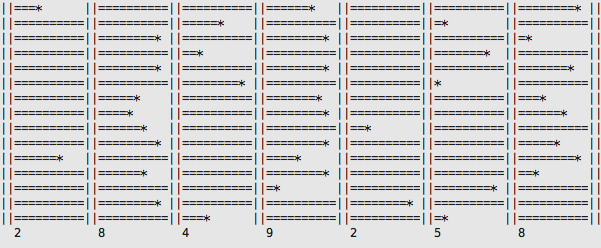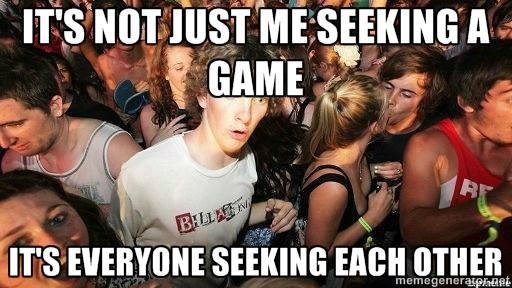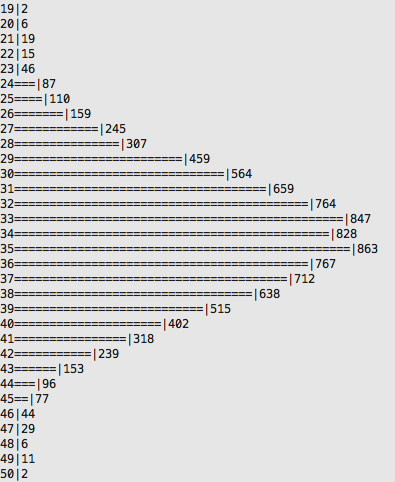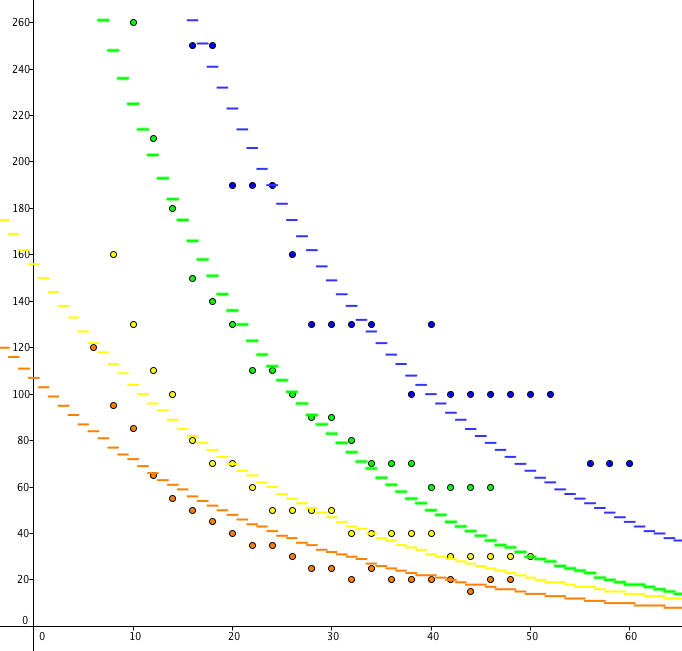# Pools and Wave Pairings

James 'Clarkey' ClarkeTechnical

The maths and science of pairing playersNobody likes waiting times, and nobody likes bad pairings; and with a finite amount of players it can very difficult to find a balance between the two.

I'll start with an example. Imagine you're trying to find an opponent; it's 6pm and you just want a decent game before it gets too late. At what point do you settle on an opponent and start playing?

Now imagine 10, 20, 30+ people all doing that at once. It's not just you who's looking for an opponent with your own set of criteria, it's everyone else seeking an opponent as well. Perhaps for them it's 2 pm and time isn't stressing them, so they're looking for a great game. Or perhaps it's 1 am and they're just jonesing for an opponent and all they want to do it play right at this very instant.As you can see, there's no clear solution. So I'm not here to tell you the solution, I'm here to explain my solution and how it mitigates some issues with other pairing methods.

## Solution 1 (Initial Solution)

Solution 1 was very rudimentary (as the system should be):

• If there are very few players in the pool, wait for 2 players and pair them immediately.
• If there's a medium amount of players in the pool, wait for 4.
• And if there's any more, wait for 6.

The issues with this system are:

• Waiting for players in this format will land you with the same opponent very frequently.
• The wait times are unpredictable.
• The Swiss-esque pairing system cannot become effective.

## Solution 2 (Wave Pairings)

This solution took several days of research, simulation, data analysis, and number crunching to develop.

Goals of this system:

• Generate good pairings in a minimum amount of time.
• Make wait times predictable, and structured.
• Allow for a rest period between games.

Let's start by developing definitions for some of these terms. 'Good pairings' occur when the Swiss-esque ranking method can operate to some capacity. 2 players is far too little (on average), 4 players is closer but still not a good sample size, and 6 players seems like just enough to keep the pairings fresh.
I then decided that 'minimum time' would be the lowest amount of time to achieve 6 player pairings on average (with upper and lower bounds when there are very few, or very many players).

### Research and Investigation

I started my investigation by inspecting the average game lengths of 1+0 games, as well as their Standard Deviation. I discovered that the average 1+0 game length was 100 seconds with a deviation of 20 seconds.

My initial impression was that the period between pairings would simply be a number that evenly divided into the average length of a game. If there were 12 players, I'd make the wavelength 100/2 = 50 seconds to divide the players into two groups of 6. This wasn't far from the truth, but it is not a well based or substantiated solution.

### Simulation

Next, I began simulating games and pairing them in waves. A game's length is defined as 100 seconds (average) + a random number between -20 and +20 (deviation) + a random number between 0 and 15 (idle). Idle is the amount of time after a game that a player remains on the game page, or desiring to do nothing.Image above: Each row represents a game; each column '||' represents a wave; '=' resepresents the game is in session; '*' represents the conclusion of a game; and the number below each column represents the amount of games that have ended in the above wave.

### Data Analysis

The next stage was to analyse how the wavelength affected the distribution of the amount of pairings made each round.Image above: It turns out that with a fixed wavelength and a fixed amount of players, the amount of pairings made each round is an ideal bellcurve. (200 players, 45 second wave length, 10 000 waves)

### Final Number Crunching

Now that we know how to find the mode amount of pairings made each wave as a function of: players, average game length and deviation, desired idle time, and wavelength; we can idealise the mode (most frequent amount of pairings) to pair 6 players each wave. The above process was then repeated for 3+0, 5+0, and 5+5 time controls.

Plotting these values ( X-axis: Amount of players, Y-axis: Wavelength to achieve 6 player pairings ) I discovered the graphs below.Image above: Red: 1+0 data plot with ideal curve fitting; Yellow: 3+0; Green: 5+0; Blue: 5+5.

### Results

Ideal wavelengths:

• 1+0 : 106.72 * exp ( -0.04 * x )
• 3+0 : 155.65 * exp ( -0.04 * x )
• 5+0 : 370.29 * exp ( -0.05 * x )
• 5+5 : 495.45 * exp ( -0.04 * x )

Where x is the amount of players currently active within the pool, and exp() is the natural exponential function.

However these are the ideal timings, some caveats must be made.

When there are few players, regardless of how mathematically optimal is it to have 2 minute wavelengths, people are simply not that patient (especially if you're the odd one out who has to wait for the next round of pairings). Because of this, in the 1+0 pool there is an upper limit of 60 seconds, and the rest of the pools have an upper limit of 80 seconds.

When there are very many players, the wavelengths tend towards 0 seconds. To give players some time between rounds, there is a lower limit of 20 second rounds.

### Conclusion

Pools work better when there are more players, try and be patient when there are few players as the wait times aren't going to be fantastic in any case.

### Pro Tip

Return to the pool as soon as you can after your game. If there's an odd amount of players to be paired, the player who has been waiting the least amount of time will need to wait until the next round to be paired.

Reconnecting